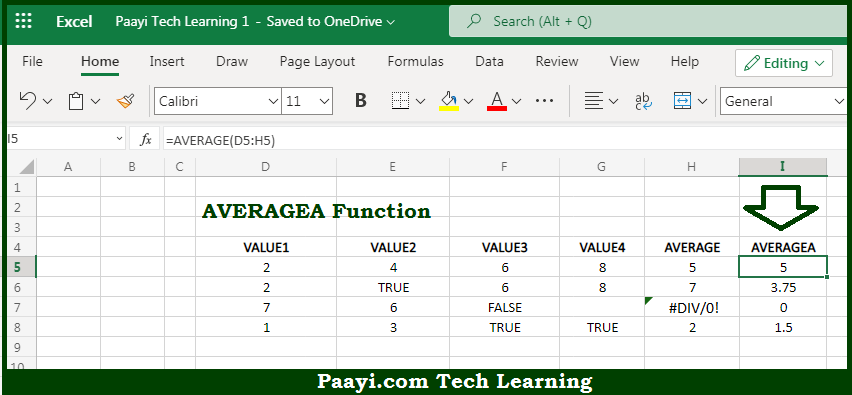# Learn How to Use Microsoft Excel AVERAGEA Function

Written by | 0 Comments | 489 Views

In this article, you will learn how to use the Microsoft Excel AVERAGEA function and its prime function in Microsoft Excel. You will also get to know the Microsoft Excel AVERAGEA function return value and syntax with the help of some examples.

Microsoft Excel AVERAGEA Function

The main purpose of the Microsoft Excel AVERAGEA function is to get the average of the group of numbers and text. That implies, with the help of AVERAGEA function you can able to calculate the average or arithmetic mean of the group of numbers provided. The AVERAGEA also evaluates the logical values TRUE and FALSE, and numbers represented as text, whereas AVERAGE just skips these values during calculation, unlike the AVERAGE function. It should be noted that the AVERAGEA function can handle 255 individual arguments, that include numbers, cell references, ranges, arrays, and constants. To calculate the average, Microsoft Excel adds the numeric value of each value together and divides it by the total number of values supplied. So, with the help of AVERAGEA function, you can able to get the average of a group of numbers provided.

Return Value of AVERAGEA Function

The return value will be the number representing an average.

Syntax of AVERAGEA Function

=AVERAGEA(value1, [value2], ...)

Where the arguments:

• value1: This is the value or reference value that can be evaluated as a number.
• value2: This is the second value or reference value that can be evaluated as a number (Optional).

## How to Use Microsoft Excel AVERAGEA Function?So we know that Microsoft Excel AVERAGEA function you can able to get the average of the group of numbers and text. That implies, with the help of AVERAGEA function you can able to calculate the average or arithmetic mean of the group of numbers provided. AVERAGEA also evaluates the logical values TRUE and FALSE, and numbers represented as text, whereas AVERAGE just skips these values during calculation, unlike the AVERAGE function.

It should be noted that the AVERAGEA function can handle 255 individual arguments, that include numbers, cell references, ranges, arrays, and constants. To calculate the average, Microsoft Excel adds the numeric value of each value together and divides it by the total number of values supplied. So, with the help of AVERAGEA function, you can able to get the average of the group of numbers provided.